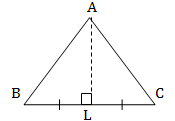### The Triangle and its Properties - Solutions 1

CBSE Class –VII Mathematics
NCERT Solutions
Chapter 6 The Triangle and its Properties
(Ex. 6.1)

Question 1. In $\mathrm{\Delta }$PQR, D is the mid-point of $\overline{\text{QR}}.$
$\overline{\text{PM}}$ is _______________
PD is ________________
Is QM = MR ?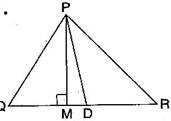$\therefore$ $\overline{\text{PM}}$ is altitude.
PD is median.
No, QM $\ne$ MR as D is the mid-point of QR.
Question 2. Draw rough sketches for the following:
1. In $\mathrm{\Delta }$ABC, BE is a median.
2. In $\mathrm{\Delta }$PQR, PQ and PR are altitudes of the triangle.
3. In $\mathrm{\Delta }$XYZ, YL is an altitude in the exterior of the triangle.
Answer: (a) Here, BE is a median in $\mathrm{\Delta }\text{ABC}$ and AE = EC.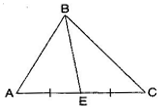(b) Here, PQ and PR are the altitudes of the $\mathrm{\Delta }$PQR and RP $\mathrm{\perp }$ QP.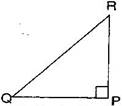(c) YL is an altitude in the exterior of $\mathrm{\Delta }$XYZ.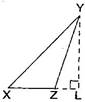Question 3. Verify by drawing a diagram if the median and altitude of a isosceles triangle can be same.
Answer: Isosceles triangle means any two sides are same.
Take $\mathrm{\Delta }\text{ABC}$ and draw the median when AB = AC.
AL is the median and altitude of the given triangle.